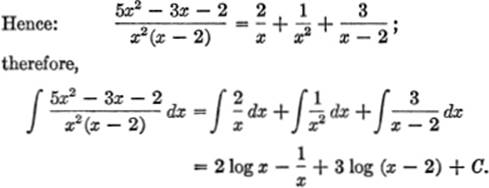﻿ ﻿INTEGRATION OF RATIONAL FRACTIONS - Special Methods of Integration - The Calculus Primer

## The Calculus Primer (2011)

### Chapter 51. INTEGRATION OF RATIONAL FRACTIONS

13—8. Rational Fractions. As used here, this term refers to a fraction in x whose numerator and denominator contain x to positive integral powers only—in other words, a fraction whose numerator and denominator are polynomials in x. For example,are rational fractions. If the degree in x of the numerator is greater than that of the denominator, as in (c) above, the fraction can be changed, by division, to the form below; thusWe shall now show how to integrate fractions of the form (a) and (b); fractions such as (c) are first reduced by division, and the resulting quotient and remainder may then be integrated.

13—9. Partial Fractions. To integrate a rational fraction, we first resolve it, by a well-known method of algebra, into partial fractions. For our purposes, we shall consider only three types, limiting the discussion to the simpler situations; other types may be dealt with by analogous methods, but are more cumbersome and occur less frequently in practice.

13—10. Case I: When the Denominator May Be Factored into Real, Linear Factors, None of Which Are Repeated. The following examples illustrate both the method of resolving the integrand into partial fractions, as well as the actual integration; the latter is, of course, a relatively simple matter.

EXAMPLE 1. FindSolution. By algebra, we may write:To find the values of A and B, we clear of fractions, obtaining:

7x + 6 = A(x + 3) + B(x) (1)

In equation (1), let x = −3; then 7(−3) + 6 = B(−3), or B = +5. Similarly, let x = 0; then 6 = 3A, or A = 2.

Hence:Therefore,= 2 log x + 5 log (x + 3) + C.

EXAMPLE 2. FindSolution. Factoring the denominator:

3x2 11x − 4 = (3x + 1)(x − 4).

Resolving into partial fractions:Clearing of fractions:

2x − 34 = A(x − 4) + B(3x + 1).

Letting x = +4, B = −2; when x = −, A = 8.EXAMPLE 3. FindSolution. By the method of partial fractions:or, 6x2 + 12x − 30 = A(xd)(x + 2) + Bx(x + 2) + Cx(x − 3). Letting x = 0, A = 5; letting x = 3, B = 4; letting x = −2, C = −3.

Hence,Therefore:NOTE. The result may be put into a more compact form by writing 5 log x + 4 log (x − 3) 3 log (x + 2) in the form

log x5 + log (x − 3)4 log (x + 2)3,

orEXERCISE 13—6

Find the following:13—11. Case II: When the Factors of the Denominator Are All of the First Degree and Some Are Repeated. This type of rational fraction will now be integrated.

EXAMPLE 1. FindSolution. LetThen, clearing of fractions:

5x2 − 3x − 2 = Ax(x − 2) + B(x − 2) + Cx2. (1)

Now, letting x = 2, we find C = 3; letting x = 0, B = 1; substituting the values of B and C so found from (1), we get A = 2.

NOTE. An alternative method for finding the values of A, B, and C is to use the method of undetermined coefficients. Accordingly, we rewrite (1) as follows:

5x2 − 3x − 2 = Ax2 − 2Ax + Bx − 2B + Cx2,

or, 5x2 − 3x − 2 = (A + C)x2 + (B − 2A)x − 2B. (2)

Equating coefficients of like powers of x, we obtain:

A + C = 5; B − 2A = −3; −2B = −2.

Solving these three simultaneous equations by the methods of elementary algebra yields A = 2, B = 1, C = 3, as before.EXAMPLE 2. FindSolution. LetThen

−2x2x − 9 = A(x + 2)(2x + 1) + B(2x + 1) + C(x + 2)2

= (2A + C)x2 + (5A + 2B + 4C)x +(2A + B + 4C).

Hence, equating coefficients of like powers of x:or A = 1; B = 5; C = −4.

Therefore,EXAMPLE 3. FindSolution. LetClearing of fractions:

− 6x2 + 9x − 2 = A(x − l)3 + Bx(x − l)2 + Cx(x − 1) + Dx, (1)

or

− 6x2 + 9x − 2 = (A + B)x3 + (−3A2B + C)x2

+ (3A + B − C + D)x − A. (2)

From (1), if x = 0, A = 2; if x = 1, D = 1; substituting these values of A and B in (2), we obtain:

−6x2 + 9x − 2 = (2 + B)x3 + (−6 − 2B + C)x2

+ (6 + BC + 1)x − 2. (3)

Equating coefficients of like powers of x in (3):

0 = 2 + B, or B = −2;

−6 = −6 − 2B + C, or C = 2B = −4.

Hence:EXERCISE 13—7

Find the following:13—12. Case III: When the Denominator Contains One or More Factors of the Second Degree, hut None of Them Repeated. The following examples show how such a fraction may be decomposed into partial fractions; note that to each non-repeated second-degree factor in the denominator of the given fraction there corresponds a partial fraction of the formwhere A, B, p, q, r = constants.

EXAMPLE 1. FindSolution. LetClear of fractions:

13x + 36 = Ax2 + 7A + 2Bx2 − 3Bx + 2Cx − 3C

= (A + 2B)x2 + (−3B + 2C)x + (7A − 3C).

Hence,

A + 2B = 0; −3B + 2C = 13; 7A − 3C = 36.

Solving, A = 6, B = −3, and C = 2;

andTherefore:EXERCISE 13—8

Find the following integrals:EXERCISE 13—9

Review

Verify the following integrations without the use of tables:15.arc sin x dx = x arc sin x ++ C

﻿